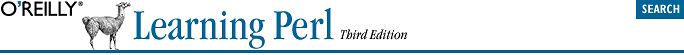home | O'Reilly's CD bookshelfs | FreeBSD | Linux | Cisco | Cisco Exam## A.3. Answers to Chapter 4 Exercises

1. Here's one way to do it:

```sub total {
my \$sum;  # private variable
foreach (@_) {
\$sum += \$_;
}
\$sum;
}```

This subroutine uses \$sum to keep a running total. At the start of the subroutine, \$sum is undef, since it's a new variable. Then, the foreach loop steps through the parameter list (from @_), using \$_ as the control variable. (Note: once again, there's no automatic connection between @_, the parameter array, and \$_, the default variable for the foreach loop.)

The first time through the foreach loop, the first number (in \$_) is added to \$sum. Of course, \$sum is undef, since nothing has been stored in there. But since we're using it as a number, which Perl sees because of the numeric operator +=, Perl acts as if it's already initialized to 0. Perl thus adds the first parameter to 0, and puts the total back into \$sum.

Next time through the loop, the next parameter is added to \$sum, which is no longer undef. The sum is placed back into \$sum, and on through the rest of the parameters. Finally, the last line returns \$sum to the caller.

There's a potential bug in this subroutine, depending upon how you think of things. Suppose that this subroutine was called with an empty parameter list (as we considered with the rewritten subroutine &max in the chapter text). In that case, \$sum would be undef, and that would be the return value. But in this subroutine, it would probably be "more correct" to return 0 as the sum of the empty list, rather than undef. (Of course, if you wished to distinguish the sum of an empty list from the sum of, say, (3, -5, 2), returning undef would be the right thing to do.)

If you don't want a possibly undefined return value, though, it's easy to remedy: simply initialize \$sum to zero rather than using the default of undef:

`my \$sum = 0;`

Now the subroutine will always return a number, even if the parameter list were empty.

2. Here's one way to do it:

```# Remember to include &total from previous exercise!
print "The numbers from 1 to 1000 add up to ", &total(1..1000), ".\n";```

Note that we can't call the subroutine from inside the double-quoted string, so the subroutine call is another separate item being passed to print. The total should be 500500, a nice round number. And it shouldn't take any noticeable time at all to run this program; passing a parameter list of 1000 values is an everyday task for Perl.

We can't do this without advanced trickiness, that is. It's rare to find anything that you absolutely can't do in Perl.